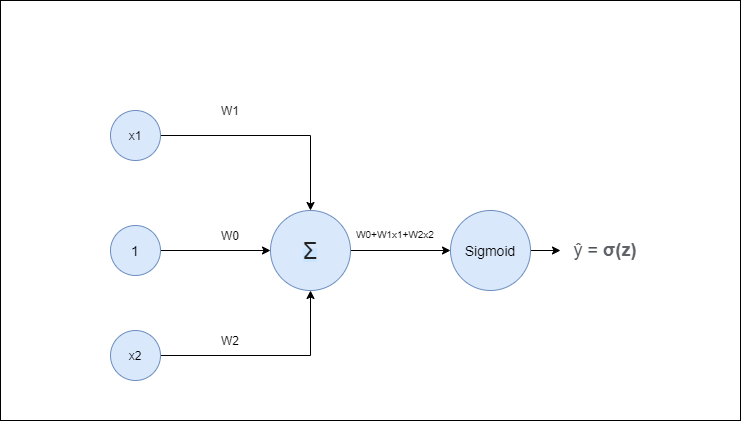# Implementing Logic Gates using Neural Networks (Part 1)

Original article was published on Artificial Intelligence on Medium# Implementing Logic Gates using Neural Networks (Part 1)

Today, I will be discussing the applications of neural networks and how they can be used as logic gates. Logic gates form the basis of any complex calculations that we perform from addition to subtraction to integration and even derivation. Logic gates such as OR, NOR, AND, NAND and XOR are prevalent and a lot of us have come across with their respective truth tables at some point in our life.

# Starting off with Neural Networks

Above is the figure of a simple neural network. Here, we have 2 input neurons or x vector having values as x1 and x2. The input neuron with value 1 is for the bias weight. The input values i.e x1, x2, and 1 are multiplied with their respective weight matrix which is W1, W2, and W0 respectively. The corresponding value is then fed to the summation neuron where we have the summed value which is

The value so obtained is fed to a neuron which has a non-linear function(sigmoid in our case) for scaling the output to a desirable range. The output range of sigmoid is 0 if the output is less than 0.5 and 1 if the output is greater than 0.5.

# Implementing OR gate

The truth table of the OR gate is as follows

The table on the left depicts the truth table of the OR gate. For the given two inputs, if any of the input is 1, the output or y is 1. The graph on the right shows the plotting of all the four pairs of input i.e (0,0), (0,1), (1,0), and (1,1). The network below is the implementation of a neural network as an OR gate.